# Strand7 linear buckling solver

The linear buckling solver calculates the buckling load factors and corresponding mode shapes for a structure under given loading conditions. It is based on the assumptions that there exists a bifurcation point where the primary and secondary loading paths intersect, and before this point is reached, all element stresses change proportionally with the load factor.

A linear buckling solution is obtained by solving the following eigenvalue problem: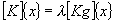where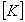Global stiffness matrix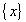Buckling mode vectors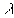Buckling load factor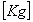Global geometric stiffness matrix

The geometric stiffness matrix, also known as the initial stress stiffness matrix, is a symmetric matrix dependent on the element stress level. It reflects the effect of geometric change on the element force vector from a known stress state. For beam and plate bending structures, the geometric stiffness matrix represents the stiffening effect of the tensile axial/membrane stresses.

The buckling solution is possible only when an existing solution is available for determining the current stress state of the structure, which is required for the calculation of the element geometric stiffness matrix. In Strand7, both linear static and nonlinear static solutions can be used to as the initial conditions for a linear buckling solution.

The linear buckling solver performs the following steps:
• Calculates and assembles the element stiffness and geometric stiffness matrices to form the global stiffness and geometric stiffness matrices. In the stiffness calculation, material temperature dependency is considered through the user nominated temperature case. Constraints are assembled in this process.

If the initial file is from a nonlinear solution, the stiffness matrix calculation is based on the current material status and geometry. In other words, a yield modulus will be used if the material has yielded and the deformed geometry will be used if the initial solution included geometry nonlinearity. In calculating stresses for the geometric stiffness matrix, the solver includes any thermal strains applied in the initial solution.

• Modifies the stiffness matrix when a shift value is applied. A shift may be used to determine modes near to a desired value.

• Solves the eigenvalue problem to get buckling load factors and the corresponding buckling modes using the Sub-Space Iteration Method.

### Notes

1. The linear buckling analysis assumes the existence of a bifurcation point where the primary and secondary loading paths intersect (point A in the figure below). At this point, more than one equilibrium position is possible. The primary path is not usually followed after loading exceeds this point and the structure is in the post-buckling state. The slope of the secondary path at the bifurcation point determines the nature of the post-buckling. A positive slope indicates that the structure will have post buckling strength whilst a negative slope means that the structure will snap through or simply collapse.Real structures have geometric and loading imperfections, often causing the primary path curve and the bifurcation point to disappear.
Another assumption for the linear buckling analysis is that the stresses in the structure increase proportionally with the load. When the deformation is large enough to disturb the stress distribution, linear buckling results will no longer be valid. In this case, a nonlinear solution is more appropriate for a realistic prediction of the structure's capacity.
1. For real structures linear buckling analysis is best used for preliminary design and studying the effects of various parameters. When the above assumptions are fully or nearly satisfied, linear buckling analysis will give accurate answers. If a more accurate estimate of the buckling load is required, it is recommended that a nonlinear analysis be carried out so that the effect of pre-buckling deformation can be included and the post buckling capacity predicted.

2. Combined linear static cases may also be used as initial conditions in the linear buckling solver.

For more information on linear buckling analysis, see Strand7 Webnotes - Linear / Statics.